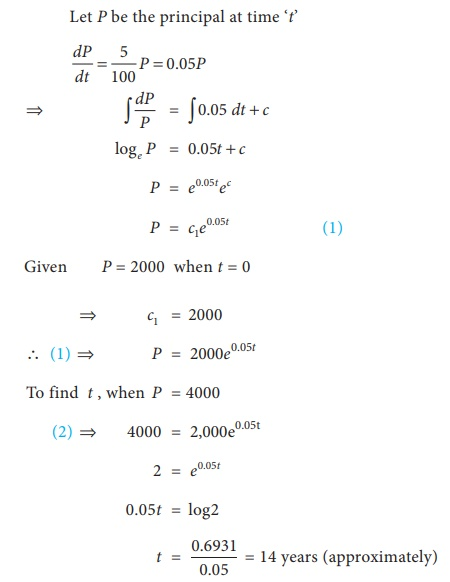Home | | Business Maths 12th Std | First order and first degree differential: Solved Example Problems

# First order and first degree differential: Solved Example Problems

Maths: Differential Equations: First order and first degree differential: Solved Example Problems

First order and first degree differential

Example 4.6

Solve: ( x2 + x +1) dx + ( y2 - y + 3)dy  = 0

Solution:Example 4.12

The marginal cost function of manufacturing x gloves is 6 + 10x  6x2 . The total cost of producing a pair of gloves is 100. Find the total and average cost function.

Solution:Example 4.13

The normal lines to a given curve at each point ( xy) on the curve pass through the point (1,0). The curve passes through the point (1,2). Formulate the differential equation representing the problem and hence find the equation of the curve.

Solution:Example 4.14

The sum of 2,000 is compounded continuously, the nominal rate of interest being 5% per annum. In how many years will the amount be double the original principal? (loge 2 = 0.6931)

Solution:Tags : with Answer, Solution, Formula | Differential Equations , 12th Business Maths and Statistics : Chapter 4 : Differential Equations
Study Material, Lecturing Notes, Assignment, Reference, Wiki description explanation, brief detail
12th Business Maths and Statistics : Chapter 4 : Differential Equations : First order and first degree differential: Solved Example Problems | with Answer, Solution, Formula | Differential Equations